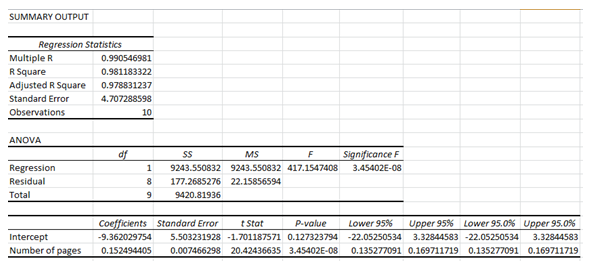# Statistics students in Oxnard College sampled 10 textbooks in the Condor bookstore, and recorded number of pages in each textbook and its cost. The bivariate data is shown below,Number of Pages (xx)Cost(yy)799117.8756073.8973135.4944160.33752101.76721109.7341351.69947136.11941131.3354970.37A student calculates a linear modelyy =  xx + . (Please show your answers to two decimal places)Use the model above to estimate the cost when number of pages is 629Cost = \$ (Please show your answer to 2 decimal places.)

Question
9 views

Statistics students in Oxnard College sampled 10 textbooks in the Condor bookstore, and recorded number of pages in each textbook and its cost. The bivariate data is shown below,

Number of Pages (xx) Cost(yy)
799 117.87
560 73.8
973 135.49
441 60.33
752 101.76
721 109.73
413 51.69
947 136.11
941 131.33
549 70.37

A student calculates a linear model

Use the model above to estimate the cost when number of pages is 629

check_circle

Step 1

Regression:

The regression analysis is conducted here by using EXCEL. The software procedure is given below:

• Enter the data.
• Select Data > Data Analysis > Regression> OK.
• Enter Input Y Range as Cost.
• Enter Input X Range as Number of pages.
• Click OK.

Step 2

The output using EXCEL is as follows:...

### Want to see the full answer?

See Solution

#### Want to see this answer and more?

Solutions are written by subject experts who are available 24/7. Questions are typically answered within 1 hour.*

See Solution
*Response times may vary by subject and question.
Tagged in